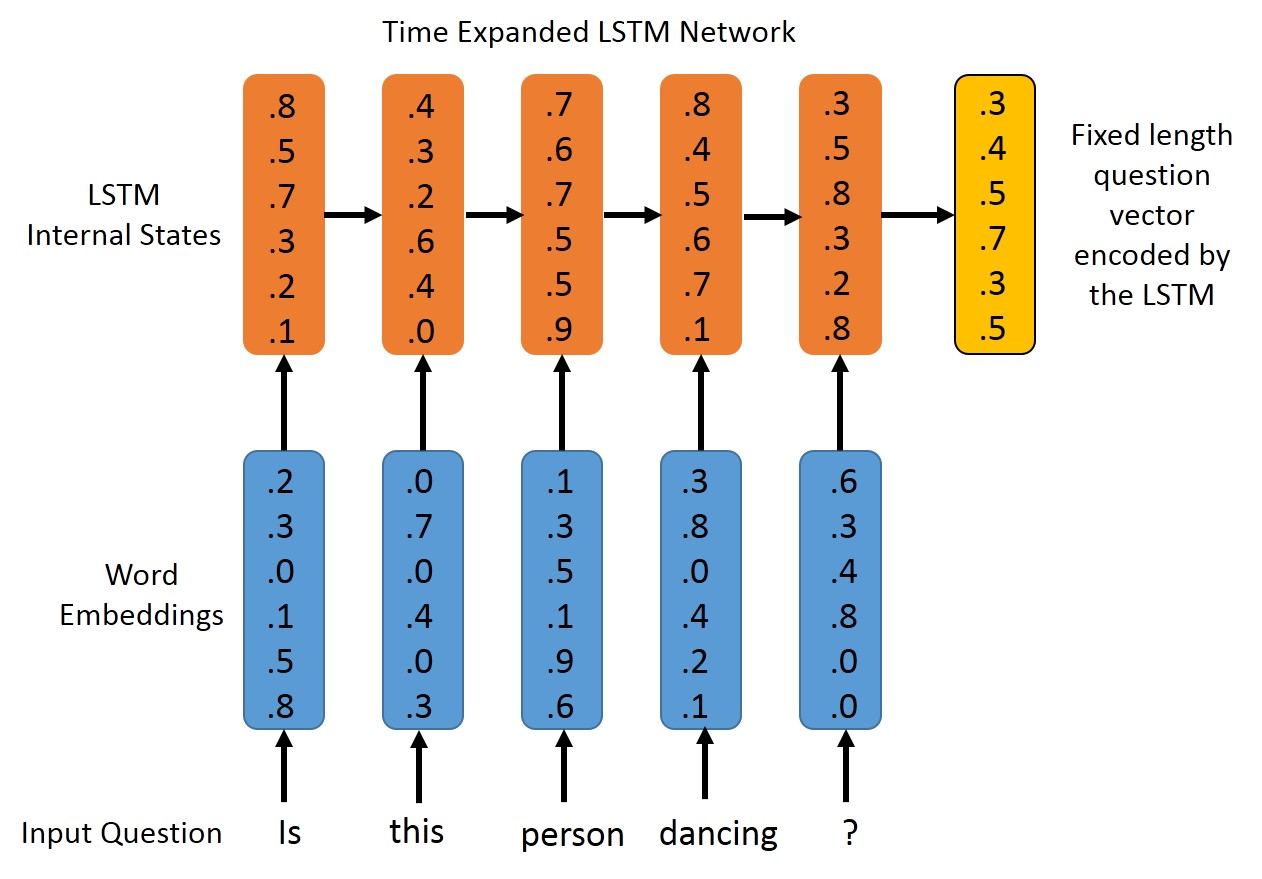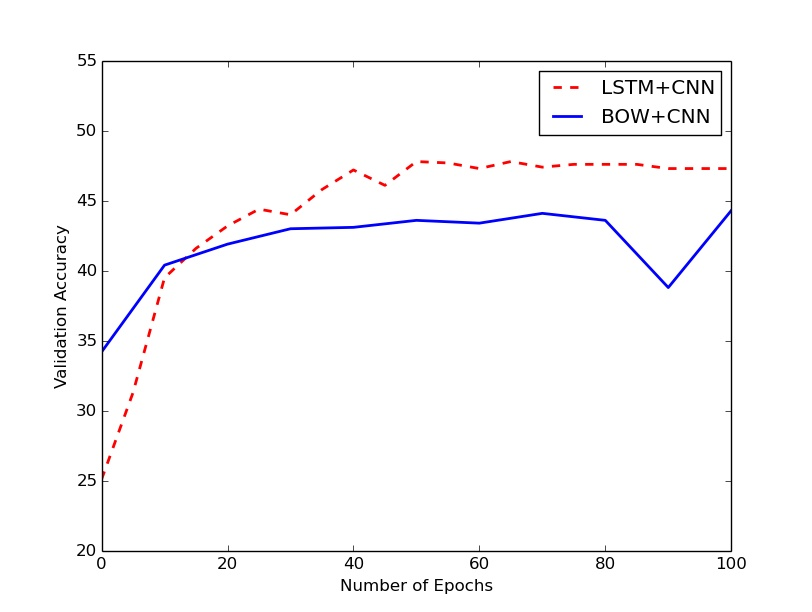Submit a blog to KDnuggets -- Top Blogs Win A Reward

Topics: AI | Data Science | Data Visualization | Deep Learning | Machine Learning | NLP | Python | R | Statistics

KDnuggets Home » News » 2015 » Nov » Tutorials, Overviews, How-Tos » Deep Learning for Visual Question Answering ( 15:n39 )

# Deep Learning for Visual Question Answering

http likes 26

Here we discuss about the Visual Question Answering problem, and I’ll also present neural network based approaches for same.

Once we have generated the feature vectors, all we need to do now is to define a model in Keras, set up a cost function and an optimizer, and we’re good to go.
The following Keras code defines a multi-layer perceptron with two hidden layers, `1024` hidden units in each layer and dropout layers in the middle for regularization. The final layer is a softmax layer, and is responsible for generating the probability distribution over the set of possible answers. I have used the `categorical_crossentropy` loss function since it is a multi-class classification problem. The `rmsprop` method is used for optimization. You can try experimenting with other optimizers, and see what kind of learning curves you get.

``````from keras.models import Sequential
from keras.layers.core import Dense, Dropout, Activation

img_dim = 4096 #top layer of the VGG net
word_vec_dim = 300 #dimension of pre-trained word vectors
nb_hidden_units = 1024 #number of hidden units, a hyperparameter

model = Sequential()
model.add(Dense(nb_hidden_units, input_dim=img_dim+word_vec_dim,
init='uniform'))
model.add(Activation('tanh'))
model.add(Dropout(0.5))
model.add(Dense(nb_hidden_units, init='uniform'))
model.add(Activation('tanh'))
model.add(Dropout(0.5))
model.add(Dense(nb_classes, init='uniform'))
model.add(Activation('softmax'))

model.compile(loss='categorical_crossentropy', optimizer='rmsprop')``````

Have a look at the entire python script to see the code for generating the features and training the network. It does not access the hard disk once the training begins, and uses about ~4GB of RAM. You can reduce memory usage by lowering the `batchSize` variable, but that would also lead to longer training times. It is able to process over 215K image-question pairs in less than 160 seconds/epoch when working on a GTX 760 GPU with a batch size of 128. I ran my experiments for 100 epochs.

### The Recurrent Neural ModelA drawback of the previous approach is that we ignore the sequential nature of the questions. Regardless of what order the words appear in, we’ll get the same vector representing the question, à la bag-of-words (BOW). A way to tackle this limitation is by use of Recurrent Neural Networks, which are well-suited for sequential data. We’ll be using LSTMs here, since they avoid some common nuances of vanilla RNNs, and often give a slightly better performance. You can also experiment with other recurrent layers in Keras, such as `GRU`. The word vectors corresponding to the tokens in the question are passed to an LSTM in a sequential fashion, and the output of the LSTM (from its output gate) after all the tokens have been passed is chosen as the representation for the entire question. This fixed length vector is concatenated with the `4096` dimensional CNN vector for the image, and passed on to a multi-layer perceptron with fully connected layers. The last layer is once again softmax, and provides us with a probability distribution over the possible outputs.

``````from keras.models import Sequential
from keras.layers.core import Dense, Activation, Merge, Dropout, Reshape
from keras.layers.recurrent import LSTM

num_hidden_units_mlp = 1024
num_hidden_units_lstm = 512
img_dim = 4096
word_vec_dim = 300

image_model = Sequential()
image_model.add(Reshape(input_shape = (img_dim,), dims=(img_dim,)))

language_model = Sequential()
language_model.add(LSTM(output_dim = num_hidden_units_lstm,
return_sequences=False,
input_shape=(max_len, word_vec_dim)))

model = Sequential()
model.add(Merge([language_model, image_model],
mode='concat', concat_axis=1))
model.add(Dense(num_hidden_units_mlp, init='uniform'))
model.add(Activation('tanh')
model.add(Dropout(0.5))
model.add(Dense(num_hidden_units_mlp, init='uniform'))
model.add(Activation('tanh')
model.add(Dropout(0.5))
model.add(Dense(nb_classes))
model.add(Activation('softmax'))

model.compile(loss='categorical_crossentropy', optimizer='rmsprop')``````

A single `train_on_batch` method call in Keras expects the sequences to be of the same length (so that is can be represented as a Theano Tensor). There has been a lot of discussion regarding training LSTMs with variable length sequences, and I used the following technique: Sorted all the questions by their length, and then processed them in batches of `128` while training. Most batches had questions of the same length (say 9 or 10 words), and there was no need of zero-padding. For the few batched that did have questions of varying length, the shorter questions were zero-padded. I was able to achieve a training speed of 200 seconds/epoch on a GTX 760 GPU.

### Show me the numbers

I trained my system on the Training Set of the VQA dataset, and evaluated performance on the validation set, following the rules of the VQA challenge. The answer produced by the Neural Net is checked against every answer provided by humans (there are ten human answers for every question). If the answer produced by the neural net exactly matches at least three of the ten answers, then we classify it as a correct prediction. Here is the performance of the models that I trained:

Model Accuracy
BOW+CNN 44.30%
LSTM-Language only 42.51%
LSTM+CNN 47.80%

It’s interesting to see that even a “blind” model is able to obtain an accuracy of 42.51%. This shows that the model is pretty good at guessing the answers once it has identified the type of question. The LSTM+CNN model shows an improvement of about 3.5% as compared to the Feedforward Model (BOW+CNN), which tells us that the temporal structure of the question is indeed helpful. These results are in line with what was obtained in the original VQA paper, albeit about 5% weaker than them. However, the results reported in the paper were on the test set (trained on train+val), while we have evaluated on the validation set (trained on only train). If we learn a model on both the training and the validation data, then we can expect a significant improvement in performance since the number of training examples will increase by 50%. Finally, there is a lot of scope for hyperparameter tuning (number of hidden units, number of MLP hidden layers, number of LSTM layers, dropout or no dropout etc.).

I carried out my experiments for 100 epochs1, and observed the following curve:The LSTM+CNN model flattens out in performance after about 50 epochs. The BOW+CNN also showed similar behavior, but took a surprising dive at epoch 90, which was soon rectified by the 100th epoch. I’ll probably re-initialize and run the models for 500 epochs, and see if such behavior is seen again or not.

#### A note on word embeddings

We have a number of choices when using word embeddings, and I experimented with three of them:

1. GloVe Word Embeddings trained on the common-crawl: These gave the best performance, and all results reported here are using these embeddings.
2. Goldberg and Levy 2014: These are the default embeddings that come with spaCy, and they gave significantly worse results.
3. Embeddings Trained on the VQA questions: I used Gensim’s word2vec implementation to train my own embeddings on the questions in the training set of the VQA dataset. The performance was similar to, but slighly worse than the GloVe embeddings. This is primarily because the VQA training set alone is not sufficiently large (~2.5m words) to get reasonable word vectors, especially for less common words.

Footnote:

• Validation was done once per 10 epochs for BOW+CNN, once every 5 epochs for LSTMs.

Bio: Avi Singh is a fourth year undergraduate at Indian Institute of Technology-Kanpur, majoring in Electrical Engineering, and pursuing a minor in Computer Science. His research interests are in Computer Vision, Machine Learning and Robotics.
github repo for this code

Related:

## Top Stories Past 30 Days

Most Popular
Most SharedGet KDnuggets, a leading newsletter on AI, Data Science, and Machine Learning

By subscribing you accept KDnuggets Privacy Policy